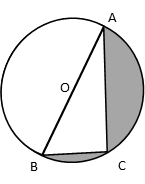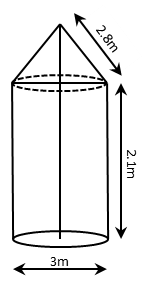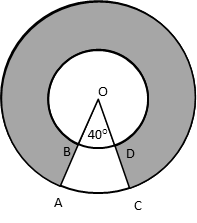# CBSE Class 10 Maths Question Paper | 2016

###### Class 10 Previous Year Question Paper Solution | 3 Mark Questions

Section C contains 10 questions of 3 marks each. Scroll down for explanatory answer and video solution to all ten 3-mark questions that appeared in Class 10 Maths CBSE board exam in 2016. Questions appeared from the following chapters : Areas Related to Circles, Surface Areas & Volumes, Coordinate Geometry, Arithmetic Progressions, Quadratic Equations, Applications of Trigonometry, and Probability.

1. O is the centre of a circle such that diameter AB = 13 cm and AC = 12 cm. BC is joined. Find the area of the shaded region. (Take π = 3.14)Hint to solve this Areas Related to Circles Question

Step 1: Because AB is a diameter, ▵ ACB is a right triangle.
Step 2: Using Pythagoras Theorem compute length of BC.
Step 3: Area of shaded region = Area of semicircle whose diameter is 13 - area of right triangle ACB.

2. A tent is in the shape of a cylinder surmounted by a conical top of same diameter. If the height and diameter of cylindrical part are 2.1 m and 3 m respectively and the slant height of conical part is 2.8 m, find the cost of canvas needed to make the tent if the canvas is available at the rate of ` 500/sq.metre. (Use π = $$frac{22}{7}$)Hint to solve this Surface Areas & Volumes problem Step 1: Cost of canvas = Area of canvas × Cost per sqm. Step 2: Area of canvas = CSA of cylinder + CSA of cone 3. If the point P$x, y) is equidistant from the points A(a + b, b – a) and P(a – b, a + b). Prove that bx = ay.

Hint to solve this Coordinate Geometry problem

Concept: Distance Formula

Step 1: Compute the length of the line segment PA using the distance formula.
Step 2: Compute the length of the line segment PB using the distance formula.
Step 3: Because P is equidistant from A and B, equate PA and PB. Essentially, equate PA2 and PB2.

4. Find the area of the shaded region, enclosed between two concentric circles of radii 7 cm and 14 cm where ∠AOC = 40°. (Use π = $$frac{22}{7}$.)Hint to solve this Areas Related to Circles problem Step 1: The shaded region is a circular disc that subtends an angle of 320° at the centre of the circle. Step 2: Area of circular disc = Area of outer circle - Area of inner circle. Step 3: 320/360 × area of circular disc is the area of the shaded region. 5. If the ratio of the sum of first n terms of two A.P's is$7n + 1) : (4n + 27), find the ratio of their mth terms.

Hint to solve this Arithmetic Progressions problem

Step 1: Write the sum of the nth term of the two APs and equate the ratio to the ratio given in the question.
Step 2: Express ratio of the mth term of the two APs.
Step 3: Compare the two ratios and express n in terms of m. Substitute 'n' in terms of m in the first ratio to arrive at the answer.

6. Solve for x : $$frac{1}{$x-1$(x-2)}) + $$frac{1}{$x-2$(x-3)}) = $$frac{2}{3}$. x ≠ 1, 2, 3. Hint to solve this Quadratic Equations problem Step 1: Take$x - 1)(x - 2)(x - 3) as common denominator of the LHS of the expression and simplify the numerator of the resultant expression.
Step 2: Cross multiply and simplify terms to get a quadratic equation.
Step 3: Solve the quadratic equation to compute the value of x.

7. A conical vessel, with base radius 5 cm and height 24 cm, is full of water. This water is emptied into a cylindrical vessel of base radius 10 cm. Find the height to which the water will rise in the cylindrical vessel, (Use π = $$frac{22}{7}$) Hint to solve this Surface Areas & Volume problem Concept: Volume of water in the conical vessel = Volume of water emptied into the cylindrical vessel. Step 1: Assign variable 'h' for the increase in height of water in the cylindrical vessel and solve for h. 8. A sphere of diameter 12 cm, is dropped in a right circular cylindrical vessel, partly filled with water. If the sphere is completely submerged in water, the water level in the cylindrical vessel rises by 3$\frac{5}{9}$ cm. Find the diameter of the cylindrical vessel. Hint to solve this Surface Areas & Volumes problem Concept: Volume of sphere dropped = Volume of water displaced in cylindrical vessel. Step 1: Assign variable 'r' for the radius of the cylindrical vessel. Step 2: Equate the two volumes. One equation, one variable. Solve for 'r' to arrive at the answer. 9. A man standing on the deck of a ship, which is 10 m above water level, observes the angle of elevation of the top of a hill as 60° and the angle of depression of the base of hill as 30°. Find the distance of the hill from the ship and the height of the hill. Hint to solve this Applications of Trigonometry problem Step 1: Applying trigonometric ratio tan the angle of depression, compute the distance between the ship and the hill Step 2: Applying tan of the angle of elevation, compute the height of the portion of the hill above the deck of the ship. Step 3: Finally compute height of hill. 10. Three different coins are tossed together. Find the probability of getting$i) exactly two heads
(iii) at least two tails.

Hint to solve this Probability problem

Step 1: The denominator for all 3 parts is the same = 8 outcomes.
Step 2: Compute the number of outcomes in which exactly two heads appear to find the answer for part i.
Step 3: Part ii) What does at least 2 heads mean? 2 or 3 heads.
Step 4: Part iii) What does at least 2 tails mean? 2 or 3 tails.

###### Try CBSE Online CoachingClass 10 Maths

Register in 2 easy steps and
Start learning in 5 minutes!